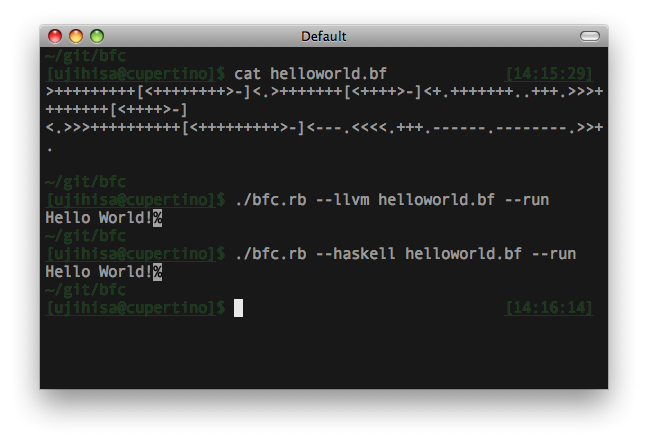# Standards

Blogged by Ujihisa. Standard methods of programming and thoughts including Clojure, Vim, LLVM, Haskell, Ruby and Mathematics written by a Japanese programmer. github/ujihisa

## Sunday, December 27, 2009

### BFC: Brainf\*\*k Compilers

Today I released BFC 1.0!

BFC: Brainf**k Compilers http://github.com/ujihisa/bfc

`bfc.rb` is a compiler written in Ruby, which can compile BF code to Ruby, C, Haskell, Scheme and LLVM.## USAGE OF BFC

``````\$ ./bfc.rb --help
\$ ./bfc.rb [-v|--version]

\$ ./bfc.rb [-r|--ruby]    helloworld.bf > helloworld.rb
\$ ./bfc.rb [-c|--c]       helloworld.bf > helloworld.c
\$ ./bfc.rb [-h|--haskell] helloworld.bf > helloworld.hs
\$ ./bfc.rb [-l|--llvm]    helloworld.bf > helloworld.ll
\$ ./bfc.rb [-s|--scheme]  helloworld.bf > helloworld.scm

\$ cat helloworld.bf | ./bfc.rb --ruby
\$ ./bfc.rb [-r|--ruby|-c|--c|-h|--haskell|-l|--llvm] helloworld.bf --run
\$ ./bfc.rb [-c|--c] helloworld.bf --without-while > helloworld.c
\$ spec ./bfc.rb
``````

## THE BRAINF**K LANGUAGE

According to Wikipedia, the programming language Brainf**k has the following 8 tokens that each have semantics. Here is the equivalent transformation from Brainf**k to C.The `bfc.rb` converts BF codes to each languages mostly based on the table.

C Translation Table in `bfc.rb`:

``````',' => '*h=getchar();',
'.' => 'putchar(*h);',
'-' => '--*h;',
'+' => '++*h;',
'<' => '--h;',
'>' => '++h;',
'[' => 'while(*h){',
']' => '}'
``````

Ruby Translation Table in `bfc.rb`:

``````',' => 'a[i]=STDIN.getc.ord',
'.' => 'STDOUT.putc(a[i])',
'-' => 'a[i]-=1',
'+' => 'a[i]+=1',
'<' => 'i-=1',
'>' => 'i+=1',
'[' => 'while a[i]!=0',
']' => 'end'
``````

They are straightforward enough not to be explained the detail.

In the same way, we can write translation tables for most programming languages except special languages including Haskell and Assembly languages.

## TRANSLATING TO HASKELL

Translating BF to Haskell needs two tricks. Haskell was difficult to handle BF because:

• Variables in Haskell are not allowed to be re-assigned
• `++h` is impossible
• There's no feature like `while` statement

So I used IO Monad with biding same-name variables, and defined `while` function.

Haskell Translation Table in `bfc.rb`:

``````',' => 'tmp <- getChar; h <- return \$ update (\_ -> ord tmp) i h;',
'.' => 'putChar \$ chr \$ h !! i;',
'-' => 'h <- return \$ update (subtract 1) i h;',
'+' => 'h <- return \$ update (+ 1) i h;',
'<' => 'i <- return \$ i - 1;',
'>' => 'i <- return \$ i + 1;',
'[' => '(h, i) <- while (\(h, i) -> (h !! i) /= 0) (\(h, i) -> do {',
']' => 'return (h, i);}) (h, i);'
``````

And the definition of `while` is:

``````while cond action x
cond x    = action x >>= while cond action
otherwise = return x
``````

This is short, but can handle loop with changing the value with larger scope like C's.

## TRANSLATING TO C WITHOUT WHILE STATEMENTS

Unlike the effort on Haskell, it is impossible to write simple translation table for C when I can use only `goto` for control flows instead of `while` statements. So I made the compile to have label counters to make labels for `goto` a lot.

Excerpt from `bfc.c`:

``````when ','; '*h=getchar();'
when '.'; 'putchar(*h);'
when '-'; '--*h;'
when '+'; '++*h;'
when '<'; '--h;'
when '>'; '++h;'
when '['; "do#{counter += 1}:"
when ']'
"if (*h != 0) goto do#{counter}; else goto end#{counter};" <<
"end#{counter}:"
end
``````

## TRANSLATING TO LLVM

LLVM Assembly language is similar to Haskell to the extent of the prohibition of re-assignments, and not similar to Haskell to the extend of having `do` syntax for Monad. So I decided to use pointers to store values. Also, LLVM needs many temporary variables which cannot be re-assigned, so I used counters again to use temporary constants.

The translation table with counters is too big to paste here, so I'll just show the definition of `'+'` which means `'++h'` in C.

``````when '+'
a = tc += 1; b = tc += 1; c = tc += 1; d = tc += 1
"%tmp#{a} = load i32* %i, align 4\n" <<
"%tmp#{b} = getelementptr [1024 x i8]* %h, i32 0, i32 %tmp#{a}\n" <<
"%tmp#{c} = load i8* %tmp#{b}, align 1\n" <<
"%tmp#{d} = add i8 1, %tmp#{c}\n" <<
"store i8 %tmp#{d}, i8* %tmp#{b}, align 1\n"
``````

(where `tc` is the abbreviation of `tmp counter`.)

One more thing. LLVM is famous for its aggressive optimizations. For example, the result of the conversion from `helloworld.bf` to LLVM Assembly Language is very long.

``````\$ ./bfc.rb --llvm ./helloworld.bf | wc -l
2842
``````

But once you optimize the assembly by `opt` command of LLVM, the line of code will become shorter and more succinct.

## SUMMARY

BFC supports compiling BF to the following language.

• Ruby
• C
• Haskell
• LLVM Assembly Language
• Scheme

In some languages it was easy to write the translator, but Haskell and LLVM was tough for me.

If I have a plenty of time, I'd like to try these challenges:

• Compiling to Erlang
• Compiling to IA-32 Assembly Language
• Compiling to LLVM Bitcode
• More Spec!
• Benchmark Suite

Anyway, I recommend you to take a look at the BFC. Enjoy!

#### 2 comments:

1.s/Excerpt from bfc.c:/Excerpt from bfc.rb:/
(in TRANSLATING TO C WITHOUT WHILE STATEMENTS)

2.